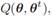# Suppose we seek to estimate θ describing a multidimensional distribution from data D, some of whose points are missing features. Consider an iterative algorithm in which the maximum likelihood value of the missing values is calculated, then assumed to be correct for the purposes of restinating θ and iterated. (a) Is this always equivalent to an Expectation-Maximization algorithm, or just a generalized Expectation-Maximization algorithm? (b) If it is an Expectation-Maximization algorithm, what is  as described by Eq. 78?

Question
1 views

Suppose we seek to estimate θ describing a multidimensional distribution from data D, some of whose points are missing features. Consider an iterative algorithm in which the maximum likelihood value of the missing values is calculated, then assumed to be correct for the purposes of restinating θ and iterated.

(a) Is this always equivalent to an Expectation-Maximization algorithm, or just a generalized Expectation-Maximization algorithm?

(b) If it is an Expectation-Maximization algorithm, what isas described by Eq. 78?

### This question hasn't been answered yet.